# A number 4

A number is made up of two digits. The sum of the digits is 11. if the digits are interchanged, the original number is increase by 9. Find the original number

x =  56

### Step-by-step explanation: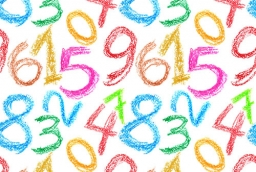Did you find an error or inaccuracy? Feel free to write us. Thank you!Tips to related online calculators
Do you have a system of equations and looking for calculator system of linear equations?

## Related math problems and questions:

• Two-digit numberIn a two-digit number, the number of tens is three greater than the number of units. If we multiply the original number by a number written in the same digits but in reverse order, we get product 3 478. Find the original number.
• Four-digit numbers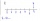Find four-digit numbers where all the digits are different. For numbers, the sum of the third and fourth digits is twice the sum of the first two digits, and the sum of the first and fourth digits is equal to the sum of the second and third digits. The di
• Two-digit numberDigit sum of thinking two-digit natural number is 11. When it exchanging a sequence of digits, given a number which is 27 less than the thinking number. Find out which number I think.
• Digit sumThe digit sum of the two-digit number is nine. When we turn figures and multiply by the original two-digit number, we get the number 2430. What is the original two-digit number?
• Two numbers 7The sum of two consecutive even numbers is 30. Find the numbers.
• The sum 16The sum of A and B is 36. The difference between A & B is 8. find the larger number of A.
• The sum 8The sum of two numbers is 21. If three times the smaller numbers is two less than twice the larger number, find the two numbers.
• Self-counting machineThe self-counting machine works exactly like a calculator. The innkeeper wanted to add several three-digit natural numbers on his own. On the first attempt, he got the result in 2224. To check, he added these numbers again and he got 2198. Therefore, he a
• Three numbersWe have three different non-zero digits. We will create all 3 digits numbers from them to use all 3 figures in each number. We add all the created numbers, and we get the sum of 1554. What were the numbers?
• Three numbersFind three numbers so that the second number is 4 times greater than the first and the third is lower by 5 than the second number. Their sum is 67.
• A five-digit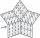A five-digit odd number has the sum of all digits (digits) five and contains two zeros. If we move each digit in the number one place to the left and move the first digit to the last place, we get a number 20,988 smaller. Find this unknown five-digit numb
• The sumThe sum of the squares of two immediately following natural numbers is 1201. Find these numbers.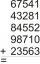Fill letters instead of digits so the indicated sum (equal letters represent equal digits). What number is hidden under the letter J? A A H A H O A H O J -------------------------- 4 3 2 1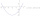The sum of of three numbers is 106. the first number is 10 more then the third. The second number is 2x the third. What are the numbers?The sum and ratio of two numbers is equal to 10. Which numbers are they?The sum of two numbers is 1. Identify this two numbers if you know that the half of first is equal to the third of second number.One number is 2 less than a second number. Twice the second number is 14 less than 5 times the first. Find the two numbers.